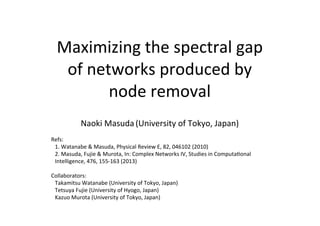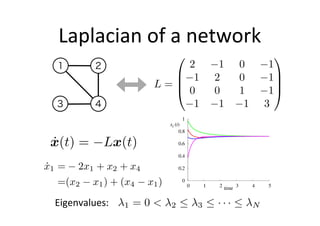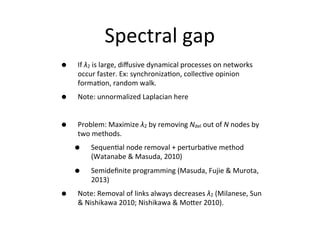Successfully reported this slideshow.

# Maximizing the spectral gap of networks produced by node removal

1

Share×
1 of 17
1 of 17

# Maximizing the spectral gap of networks produced by node removal

1

Share

Presentation slides for the following two papers (currently available in the pdf format only).

(1) T. Watanabe, N. Masuda.
Enhancing the spectral gap of networks by node removal.
Physical Review E, 82, 046102 (2010).

(2) N. Masuda, T. Fujie, K. Murota.
Semidefinite programming for maximizing the spectral gap.
In: Complex Networks IV, Studies in Computational Intelligence, 476, 155-163 (2013).

Presentation slides for the following two papers (currently available in the pdf format only).

(1) T. Watanabe, N. Masuda.
Enhancing the spectral gap of networks by node removal.
Physical Review E, 82, 046102 (2010).

(2) N. Masuda, T. Fujie, K. Murota.
Semidefinite programming for maximizing the spectral gap.
In: Complex Networks IV, Studies in Computational Intelligence, 476, 155-163 (2013).

## More Related Content

### Related Books

Free with a 14 day trial from Scribd

See all

### Related Audiobooks

Free with a 14 day trial from Scribd

See all

### Maximizing the spectral gap of networks produced by node removal

1. 1. Maximizing  the  spectral  gap   of  networks  produced  by   node  removal Naoki  Masuda  (University  of  Tokyo,  Japan) Refs:   1.  Watanabe  &  Masuda,  Physical  Review  E,  82,  046102  (2010) 2.  Masuda,  Fujie  &  Murota,  In:  Complex  Networks  IV,  Studies  in  ComputaUonal   Intelligence,  476,  155-­‐163  (2013) Collaborators: Takamitsu  Watanabe  (University  of  Tokyo,  Japan) Tetsuya  Fujie  (University  of  Hyogo,  Japan) Kazuo  Murota  (University  of  Tokyo,  Japan)
2. 2. Laplacian  of  a  network ˙x(t) = Lx(t) ˙x1 = 2x1 + x2 + x4 =(x2 x1) + (x4 x1) 1 2 3 4 L = 0 B B @ 2 1 0 1 1 2 0 1 0 0 1 1 1 1 1 3 1 C C A 1 = 0 < 2  3  · · ·  NEigenvalues:
3. 3. Spectral  gap • If  λ2  is  large,  diﬀusive  dynamical  processes  on  networks   occur  faster.  Ex:  synchronizaUon,  collecUve  opinion   formaUon,  random  walk. • Note:  unnormalized  Laplacian  here • Problem:  Maximize  λ2  by  removing  Ndel  out  of  N  nodes  by   two  methods. • SequenUal  node  removal  +  perturbaUve  method   (Watanabe  &  Masuda,  2010) • Semideﬁnite  programming  (Masuda,  Fujie  &  Murota,   2013) • Note:  Removal  of  links  always  decreases  λ2  (Milanese,  Sun   &  Nishikawa  2010;  Nishikawa  &  Mober  2010).
4. 4. PerturbaUve  method • Extends  the  same  method  for  adjacency  matrices   (Restrepo,  Ob  &  Hunt,  2008) • Much  faster  than  the  brute  force  method. Lu = 2u (L + L)(u + u) =( 2 + 2)(u + u) u = u ui ˆei where ˆei ⌘ (0, . . . , 0, 1|{z} i , 0, . . . , 0) =) 2 ⇡ P j2Ni uj(ui uj) 1 u2 i Select  i  that   maximizes  Δλ2
5. 5. Results:  model  networks (N  =  250,  <k>  =  10) Goh WS HKBA ER f 0 0.1 0.2 0.3 0.4 0.5 f 0 0.1 0.2 0.3 0.4 0.5 f 0 0.1 0.2 0.3 0.4 0.5 1 3 5 1 1.4 1.8 perturbative betweenness-based degree-based optimal sequential 1 1.2 0.9 0.8 1.1 0.9 1 1.1 1.2 1 0.6 1.4 f 0 0.1 0.2 0.3 0.4 0.5 f 2normalized 0 0.1 0.2 0.3 0.4 0.5 Goh
6. 6. Results:  real  networks perturbative betweenness-based degree-based optimal sequential e-mail C. elegans 2 3 4 5 0.5 0 1 1.5 2 22 E. coli 0 0.2 0.4 0.6 0.8 macaque 1 2 3 4 5 0 0.1 0.2 0.3 0.4 0.5 0 0.1 0.2 0.3 0.4 0.5 0 f f 0 0.1 0.2 0.3 0.4 0.5 0 0.1 0.2 0.3 0.4 0.5 f f N  =  279 <k>  =  16.4 N  =  1133 <k>  =  9.62 N  =  71 <k>  =  12.3 N  =  2268 <k>  =  4.96
7. 7. Conclusions • Careful  node  removal  can  increase  the   spectral  gap. • For  a  variety  of  networks,  the  perturbaUve   strategy  works  well  with  a  reduced   computaUonal  cost. • Ref:  Watanabe  &  Masuda,  Physical  Review   E,  82,  046102  (2010)
8. 8. However, • SequenUal  opUmal  may  not  be  opUmal  for   Ndel  ≥  2. • An  obvious  combinatorial  problem  if  we   pursue  the  opUmal  soluUon.
9. 9. min  t  subject  to tI F(x1, . . . , xn) ⌫ 0 (eigenvalues: t n  · · ·  t 1) Semideﬁnite  programming Eigenvalue  minimizaUon  using  SDP nX i=1 ciximin subject  to F0 + nX i=1 xiFi ⌫ 0 F0, . . . , Fn :  symmetric  matrices F(x1, . . . , xn) = F0 + nX i=1 xiFi (eigenvalues: 1  · · ·  n) F0, . . . , Fn :  symmetric  matrices
10. 10. DiﬃculUes  in  our  case • Discreteness:  xi  ∈  {0,  1} • Ndel  (irrelevant)  0  eigenvalues  appear. • Not  interested  in  the  zero  eigenvalue    λ1=0. • So,  let’s  start  with  the  following  problem: max  t  subject  to λ1=0  →  λ1’=α New  zero  eigenvalue  →  β But,  a  nonlinear  constraint   tI + X i<j;(i,j)2E xixj ˜Lij + ↵J + NX i=1 (1 xi)Ei ⌫ 0 NX i=1 xi = N Ndel, xi 2 {0, 1} where Ei = diag(0, . . . , 0, 1|{z} i , 0, . . . , 0) L = X 1i<jN;(i,j)2E ˜Lij
11. 11. (Lovász,  1979;  Grötschel,  Lovasz  &  Schrijver,  1986;  Lovasz  &  Schrijver,  1991) • Xij,  where  (i,j)  is  not  a  link,  is  a  “free”  variable. • We  can  reduce  the  number  of  variables  using  Xii  =  xi.  But  sUll  O(N2)  terms  exist,  and  the  algorithm  runs  slowly. • For  a  technical  reason,  we  set  α  =  β/N • Challenges • Discreteness  of  xi  →    “relax”  the  problem • Nonlinear  constraint  →    introduce  new  vars Xij ⌘ xixj tI + X i<j;(i,j)2E Xij ˜Lij + ↵J + NX i=1 (1 xi)Ei ⌫ 0 NX i=1 xi = N Ndel Y ⌘  1 x> x X ⌫ 0 0  xi(= Xii)  1(1  i  N) SDP1 ←  actually  not  needed tI + X i<j;(i,j)2E xixj ˜Lij + ↵J + NX i=1 (1 xi)Ei ⌫ 0 max  t  subject  to
12. 12. An  improved  method  SDP2:  “local  relaxaUon” tI + X i<j;(i,j)2E Xij ˜Lij + ↵J + NX i=1 (1 xi)Ei ⌫ 0 x1x2 0 x1(1 x2) 0 (1 x1)x2 0 (1 x1)(1 x2) 0 X12 0 x1 X12 0 x2 X12 0 1 x1 x2 + X12 0
13. 13. IntuiUve  comparison • Consider  N=1  (unrealisUc  though). • SDP1 • Note:  In  fact,  X11  =  x1. • SDP2 • Linear!  1 x> x X =  1 x1 x1 X11 ⌫ 0 () X11 x2 1 8 >>>< >>>: Xij 0 xi Xij 0 xj Xij 0 1 xi xj + Xij 0 with i = j = 1 =) 8 >< >: X11 0 X11  x1 X11 2x1 1
14. 14. • Number  of  vars  reduced. • Size  of  the  SDP  part  reduced. • Constraint  0  ≤  xi  ≤  1  unnecessary. SDP2 max  t  subject  to tI + X i<j;(i,j)2E Xij ˜Lij+↵J + NX i=1 (1 xi)Ei ⌫ 0, NX i=1 xi =N Ndel, For links (i, j) 8 >>>< >>>: Xij 0 xi Xij 0 xj Xij 0 1 xi xj + Xij 0
15. 15. Small  networks Karate  club (N=34,  78  links,  β=2) Data:  Zachary  (1977)   Macaque  corUcal  net (N=71,  438  links,  β=2) Data:  Sporns  &  Zwi  (2004)     0 1 2 0 10 20 2 Ndel (a) sequential SDP1 SDP2 0 1 2 3 0 10 20 2 Ndel (b)
16. 16. RelaUvely  large  networks BA  model  (scale-­‐free  net) (N=150,  297  links,  β=2) C.  elegans  neural  net (N=297,  2287  links,  β=2.5) Data:  Chen  et  al.  (2006) 0.5 0.6 0.7 0 10 20 2 Ndel (c) 1 1.5 2 2.5 3 0 10 20 30 2 Ndel (d) SDP2 sequenUal ObservaUon:  SDP1/SDP2  may  work  beber  for  sparse  networks.
17. 17. Possible  direcUons • Go  violate  convexity • (1-­‐xi)  →  (1-­‐xi)p,  and  increase  p  gradually   from  p=1.  By  the  Newton  method • Parameter  tuning? tI + X i<j;(i,j)2E Xij ˜Lij + ↵J + NX i=1 (1 xi)Ei ⌫ 0